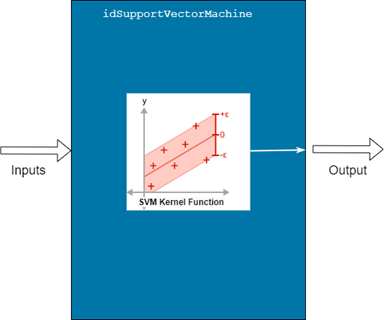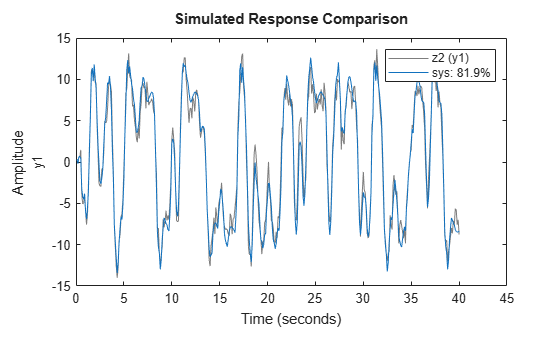# idSupportVectorMachine

Support vector machine regression mapping function for nonlinear ARX models (requires Statistics and Machine Learning Toolbox)

Since R2022a

## Description

An `idSupportVectormachine` object implements a support vector machine (SVM) regression model, and is a nonlinear mapping function for estimating nonlinear ARX models. This mapping object, which is also referred to as a nonlinearity, incorporates objects that the mapping function creates using Statistics and Machine Learning Toolbox™ functions. The mapping object contains a single component—the nonlinear component that the SVM algorithm represents.

The input to the mapping object can be a vector of the regressors of a nonlinear ARX model.Mathematically, `idSupportVectorMachine` is a function that maps m inputs X(t) = [x(t1),x2(t),…,xm(t)]T to a scalar output y(t) using the following relationship:

`$y\left(t\right)=S\left(Χ\left(t\right)\right)$`

Here, S(X(t)) is the regressive SVM function that constitutes the kernel of the `idSupportVectorMachine` object, and can be expressed as:

`$S\left(X\right)=\sum _{n=1}^{N}{\alpha }_{n}G\left({x}_{n},X\right)+b$`

Here:

• X is an m-by-1 vector of inputs, or regressors.

• N is the number of support vectors in the trained model.

• xn is the nth support vector in the trained model.

• αn is the weight associated with each support vector.

• G is the Gram matrix that results from the operation of the specified kernel function on X and xn.

• b is the offset of the trained model.

The SVM mapping object implements linear epsilon-insensitive SVM regression, which is particularly robust to outliers. In this type of regression, a primary goal of the algorithm is to find a function f(x) that deviates from a training response yn by a value no greater than ε for each training point. The algorithm accommodates situations where no such linear function is available by introducing “slack variables” ζ and ζ* that provide a softer margin beyond ε. The corresponding loss function, known as the ε-insensitive loss function, ignores observed values that are within ε by treating them as equal to 0. The loss, Lε, is based only on the distance between the observed value y and the ε boundary when the value is beyond that boundary, as described by:

For more information about SVM regression models, see Understanding Support Vector Machine Regression (Statistics and Machine Learning Toolbox).

Use `idSupportVectorMachine` as the value of the `OutputFcn` argument of an `idnlarx` model. For example, specify `idSupportVectorMachine` when you estimate an `idnlarx` model with the following command.

`sys = nlarx(data,regressors,idSupportVectorMachine)`

You can configure the `idSupportVectorMachine` object to fix parameters during estimation and modify options. For example, if you are using a previously estimated `idSupportVectorMachine` model `S` and want to retain the model parameters, fix the parameters during `idnlarx` estimation by setting the `S.Free` property to `false`. To modify an estimation option, set the value of the option property in `S.EstimationOptions`. For example, to change the solver to `'ISDA'`, use the command `S.EstimationOptions.Solver = 'ISDA'`. Use `evaluate` to compute the output of the function for a given vector of inputs.

## Creation

### Syntax

``S = idSupportVectorMachine``
``S = idSupportVectorMachine(KernelFunction)``
``S = idSupportVectorMachine(KernelFunction,EpsilonMargin)``

### Description

example

````S = idSupportVectorMachine` creates an `idSupportVectorMachine` object `S` with the kernel function `'Gaussian'` and default kernel parameters. The number of inputs is determined during model estimation and the number of outputs is 1.```
````S = idSupportVectorMachine(KernelFunction)` specifies the specific kernel `KernelFunction` .```
````S = idSupportVectorMachine(KernelFunction,EpsilonMargin)` specifies the property `EpsilonMargin`, which is half the width of the epsilon-insensitive band. ```

## Properties

expand all

Input signal names for the inputs to the mapping object, specified as a 1-by-m cell array, where m is the number of input signals. This property is determined during estimation.

Output signal name for the output of the mapping object, specified as a 1-by-1 cell array. This property is determined during estimation.

SVM kernel function name, specified as `'Gaussian'`, `'rbf'`, `'Linear'`, or `'Polynomial'`. The SVM algorithm uses the kernel function to compute the Gram matrix.

Kernel scale parameter, specified as `'auto'` or a positive scalar. The software divides elements of the predictor matrix by this value prior to computing the Gram matrix. `KernelScale` is an important tuning parameter for SVM models.

When you specify `'auto'`, the software uses the `fitrsvm` `'auto'` setting.

Half the width of the epsilon-insensitive band, specified as `'auto'` or a nonnegative scalar. For more information on this band, see Understanding Support Vector Machines (Statistics and Machine Learning Toolbox).

Polynomial kernel function order, specified as a positive integer that the software uses when `KernelFunction` is `'Polynomial'`.

Kernel offset order, specified as a nonnegative scalar. The software adds `KernelOffset` to each element of the Gram matrix.

Box constraint that is the upper bound for the absolute value of the `alpha` coefficients, specified as a positive scalar.

Support vector parameters of the trained SVM model, specified as shown in the following table.

ParameterDescriptionDefault
`Alpha`

Initial estimates of the α weights associated with the support vectors, specified as a numeric vector with length equal to the number of rows in the estimation data set.

You can set the values of `Alpha` only prior to estimation.

`[]`
`LinearCoefficient`Linear coefficients that the software estimates when `KernelFunction` is `'Linear'` .Read-only property
`Bias`SVM model offset term that the software estimates.Read-only property
`IsSupportVector`Logical vector with length equal to the number of observations that indicates which observations are support vectors.Read-only property

Option to free or fix SVM model parameters during estimation, specified as a logical scalar. If all the parameters have finite values, such as when the `idSupportVectorMachine` object corresponds to a previously estimated model, then setting `Free` to `false` causes the parameters of the kernel G(X) to remain unchanged during estimation. The default value is `true`.

Estimation options for the `idSupportVectorMachine` model, specified as shown in the following table. For more information on any of these options, see `fitrsvm` (Statistics and Machine Learning Toolbox).

OptionDescriptionDefault
`OutlierFraction`Expected proportion of outliers in the training data, specified as a numeric scalar in the interval `[0,1]` `[ ]`
`CacheSize`Cache size in MB that the software reserves in memory for training the model, specified as a positive scalar.`1000`
`Solver`

Solver used for parameter estimation, specified as one of the following values:

• `'SMO'` — Sequential Minimal Optimization

• `'ISDA'` — Iterative Single Data Algorithm

• `'ISDA'`, if you set `OutlierFraction` to a positive value

• `'SMO'`, otherwise

## Examples

collapse all

Load the data `z2`.

`load iddata2 z2`

Create an `idSupportVectorMachine` mapping object.

`S = idSupportVectorMachine`
```S = Support Vector Machine Function Nonlinear Function: Support Vector Machine function using a Gaussian kernel Inputs: {1x0 cell} Outputs: {1x0 cell} KernelFunction: 'Gaussian' EpsilonMargin: 'auto' PolynomialOrder: 3 KernelOffset: 0 BoxConstraint: 1 KernelScale: 'auto' Parameters: '<Support vector parameters>' Free: 1 EstimationOptions: '<Estimation option set>' ```

Set the `KernelScale` property to 5.

`S.KernelScale = 5;`

Estimate a nonlinear ARX model that uses `S` as the output function.

`sys = nlarx(z2,[4 4 1],S);`

View the properties of `sys.OutputFcn`.

`sys.OutputFcn`
```ans = Support Vector Machine Function Inputs: y1(t-1), y1(t-2), y1(t-3), y1(t-4), u1(t-1), u1(t-2), u1(t-3), u1(t-4) Output: y1(t) Nonlinear Function: Support Vector Machine function using a Gaussian kernel Inputs: {'y1(t-1)' 'y1(t-2)' 'y1(t-3)' 'y1(t-4)' 'u1(t-1)' 'u1(t-2)' 'u1(t-3)' 'u1(t-4)'} Outputs: {'y1(t)'} KernelFunction: 'Gaussian' EpsilonMargin: 1.0424 PolynomialOrder: 3 KernelOffset: 0 BoxConstraint: 1 KernelScale: 5 Parameters: '<Support vector parameters>' Free: 1 EstimationOptions: '<Estimation option set>' ```

Compare `sys` with `z2`.

`compare(z2,sys)`## Version History

Introduced in R2022a